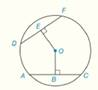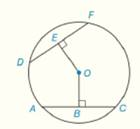Chapter 6.CR, Problem 26CRElementary Geometry For College St...

7th Edition
Alexander + 2 others
ISBN: 9781337614085

Solutions

Chapter
SectionElementary Geometry For College St...

7th Edition
Alexander + 2 others
ISBN: 9781337614085
Textbook Problem

Given :                               O E - ≅ O B -   i n   ⨀   O                                                       D F = x ( x - 2 )                                                                       A C = x + 28             F i n d :                                     D E   a n d   A CTo determine

To Find: DE and AC, If OE-OB- in  ODF=x(x-2) and AC=x+28Explanation

Concept:

Theorem 6.1.1:

A radius that is perpendicular to a chord bisects the chord.

Theorem 6.1.7:

Chords that are located at the same distance from the center of a circle are congruent.

Theorem 6.1.8:

Congruent chords are located at the same distance from the center of a circle.

Calculation:

Given that OE-OB- in  O

So, OE and OB are congruent distances.

So, by Theorem 6.1.7:

Chords that are located at the same distance from the center of a circle are congruent.

DF and AC are at the same distance from the center of a circle  O.

So, DF=AC

But it is given that, DF=x(x-2) and AC=x+28

So, x(x-2)=x+28

By distributive property, x2-2x=x+28

Subtract x+28 from each side

x2-2x-x-28=x+28-x-28

x2-3x-28=0

Factorize, x2-7x+4x-28=0

xx-7+4

Still sussing out bartleby?

Check out a sample textbook solution.

See a sample solution

The Solution to Your Study Problems

Bartleby provides explanations to thousands of textbook problems written by our experts, many with advanced degrees!

Get Started

Find more solutions based on key concepts